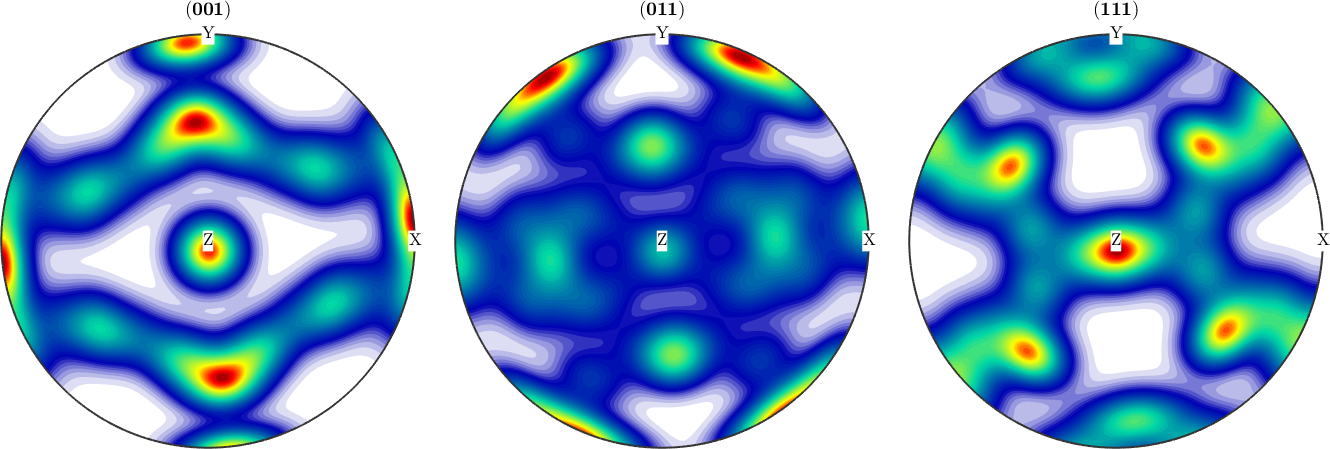centerSpecimen edit page

rotates an odf with specimen symmetry into its symmetry axes

centerSpecimen(odf,center) trys to find the normal vectors of orthorhombic symmetry for the x mirror and y mirror plane and calculates an rotation needed to rotate the odf back into these mirror planes. the routine starts with an lookaround grid for a given center (default xvector) to find a starting value for newton iteration.

## Input

 odf SO3Fun v0 vector3d initial gues for a symmetry axis (default xvector)

## Output

 odf rotated SO3Fun rot rotation such that rotate(odf_out,r) = odf_in v1,v2 normal vector of the two fold symmetry axes

## Options

 SO3Grid a SO3Grid where the SO3Fun is evaluated on delta specifies the opening angle for the initial search grid around input center resolution specifies the resolution for the initial search grid silent dont verbose number of initial axes and the newton iteration fourier use fourier coefficents as objective function

## Example